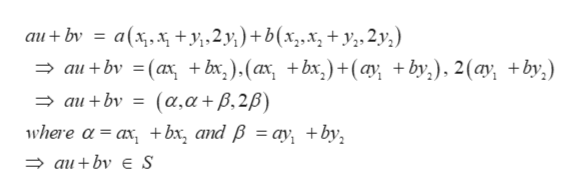# Consider the set ? = ((x, x+ y, 2y)|x,y are real) of vectors in r3 . Is W a subspace? If yes, prove it and if no explain the reason.

Question
32 views

Consider the set ? = ((x, x+ y, 2y)|x,y are real) of vectors in r3 . Is W a subspace? If yes, prove it and if no explain the reason.

check_circle

Step 1

We have Set S = {(x, x + y, 2y) | x, y are real}. Let u, v be any two element of S then,

Step 2

Let a and b be any two element of R. In order to prove that S is a subspace of V, we have to prove that au + bv S, for which we have to show that au + bv is expressible in the form ...help_outlineImage Transcriptionclosea(xxy2)+ b(x,.x, +y,2y,) - aι+bν - (α + bx ) (α + bx) +(ωγ +by ) 2 (α + bv) au+ bν ( α,α + β, 2β) where α- a, + bx, and βa) +bν, Φau + bν au+bν E S fullscreen

### Want to see the full answer?

See Solution

#### Want to see this answer and more?

Solutions are written by subject experts who are available 24/7. Questions are typically answered within 1 hour.*

See Solution
*Response times may vary by subject and question.
Tagged in

### Math